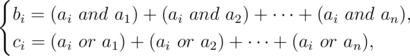# CF734F Anton and School

• 26通过
• 45提交
• 题目来源
• 评测方式 RemoteJudge
• 标签
• 难度 省选/NOI-
• 时空限制 2000ms / 256MB
• 提示：收藏到任务计划后，可在首页查看。

## 题意翻译

题目描述
Anton在学校最喜欢的课程是数学研究。他通常很快就完成了所有的任务，但这此老师给了他一个很复杂的任务：给出两个长度为n的矩阵b和c，找到数组a，这样：
b[i]=(a[i] and a)+(a[i] and a)+...+(a[i] and a[n]),
c[i]=(a[i] or a)+(a[i] or a)+...+(a[i] or a[n]),
其中a and b表示按位与，a or b表示按位或。

通常Anton在数学研究方面表现不错，但是这个问题太难了，所以Anton要求你帮忙。

## 题目描述

Anton goes to school, his favorite lessons are arraystudying. He usually solves all the tasks pretty fast, but this time the teacher gave him a complicated one: given two arrays $b$ and $c$ of length $n$ , find array $a$ , such that:where $a and b$ means bitwise AND, while $a or b$ means bitwise OR.

Usually Anton is good in arraystudying, but this problem is too hard, so Anton asks you to help.

## 输入输出格式

输入格式：

The first line of the input contains a single integers $n$ ( $1<=n<=200000$ ) — the size of arrays $b$ and $c$ .

The second line contains $n$ integers $b_{i}$ ( $0<=b_{i}<=10^{9}$ ) — elements of the array $b$ .

Third line contains $n$ integers $c_{i}$ ( $0<=c_{i}<=10^{9}$ ) — elements of the array $c$ .

输出格式：

If there is no solution, print $-1$ .

Otherwise, the only line of the output should contain $n$ non-negative integers $a_{i}$ — elements of the array $a$ . If there are multiple possible solutions, you may print any of them.

## 输入输出样例

输入样例#1： 复制
4
6 8 4 4
16 22 10 10

输出样例#1： 复制
3 5 1 1

输入样例#2： 复制
5
8 25 14 7 16
19 6 9 4 25

输出样例#2： 复制
-1

提示
标程仅供做题后或实在无思路时参考。
请自觉、自律地使用该功能并请对自己的学习负责。
如果发现恶意抄袭标程，将按照I类违反进行处理。